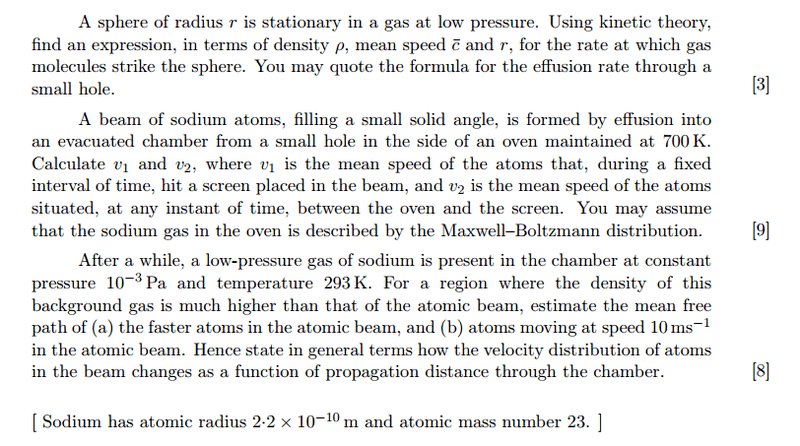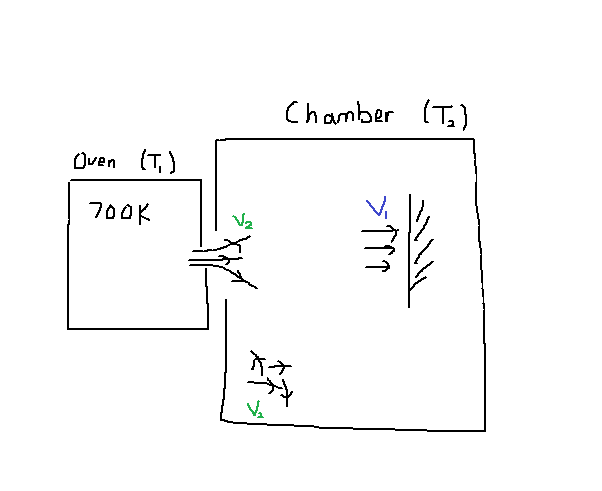# Effusion and speed of molecules

unscientific

## Homework StatementPart(a): Find rate of incidence of gas molecules.
Part(b): Find v1 and v2.
Part (c): Find mean free path for faster molecules.
Part (d): Find mean free path of 10m/s molecules.

## The Attempt at a Solution

Part (a)

Letting ##\phi## be flux, and ##\Delta p## be change in momentum of rebounding molecule,
$$\phi \Delta p = \left(\frac{4}{3}\pi r^3\right)\rho g$$
$$\phi (2m\overline c) = \left(\frac{4}{3}\pi r^3\right)\rho g$$
$$R = \frac{2}{3}\frac{r\rho g}{m \overline c}$$

Funny how the mass of the molecule is not given. But this make sense as the more massive the atom traveling at a certain speed, the less flux you need to support the weight.

Part (b)The speed distribution of molecules coming out of the oven is given by ##f = 2\alpha^2 v^3 e^{-\alpha v^2}##.

$$v_2 = \int_0^{\infty} v f dv$$
$$= \frac{3}{4}\sqrt{\frac{\pi}{\alpha}}$$
$$= \sqrt{\frac{9}{8}\frac{\pi kT_1}{m}}$$

For interest, let's calculate the temperature of the Chamber:

$$v_2 = \sqrt{\frac{8kT_2}{\pi m}} = \sqrt{\frac{9}{8}\frac{\pi kT_1}{m}}$$
$$T_2 = \left(\frac{3}{8}\pi\right)^2 T_1\approx 972K$$

To calculate ##v_1##, we use the speed distribution of the chamber (not the one coming out of the oven!)

$$g = 2\alpha_2^2 v^3 exp(-\alpha_2 v^2)$$

$$v_1 = 2\alpha_2^2 \int_0^{\infty} v^4 exp(-\alpha_2 v^2)$$
$$= \sqrt{\frac{9}{8}\frac{\pi kT_2}{m}}$$
$$= \pi \sqrt{\frac{81}{512}\frac{\pi k T_1}{m}} \approx 1112 ms^{-1}$$

Part (c)
I'm not sure whether the mean free path calculated here is correct.

The relative speed is ##v_r = |v_2 - v_1| = 1112 - 944 = 168 ms^{-1}## and ##n = \frac{P}{kT} = 2.47*10^{17}## and ##\sigma = 1.52*10^{-19}##.

Mean free path = (Distance travelled)/(No. of collisions during that distance)

$$\lambda = \frac{v_2t}{v_r n \sigma t} = 176m$$

Part (d)
In this case, ##v_r = |1112 - 10| = 1102##.

$$\lambda = \frac{10t}{v_r n \sigma t} = 0.24 m$$

This is roughly 700 times less than above.

This means that the atoms in the beam have a much larger mean free path than the atoms in the chamber. Thus velocity of the beam reduces much only after 176m into its flight path.